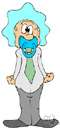# regression

(redirected from Regression function)
Also found in: Thesaurus, Medical, Legal, Financial, Encyclopedia.
Related to Regression function: Population regression function

## re·gres·sion

(rĭ-grĕsh′ən)
n.
1. The process or an instance of regressing, as to a less perfect or less developed state.
2. Psychology Reversion to an earlier or less mature pattern of feeling or behavior.
3. Medicine Subsidence of the symptoms or process of a disease.
4. Statistics A technique for predicting the value of a dependent variable as a function of one or more independent variables in the presence of random error.
5. Astronomy Retrograde motion of a celestial body.
6. Geology A relative fall in sea level resulting in deposition of terrestrial strata over marine strata.

## regression

(rɪˈɡrɛʃən)
n
1. (Psychology) psychol the adoption by an adult or adolescent of behaviour more appropriate to a child, esp as a defence mechanism to avoid anxiety
2. (Statistics) statistics
a. the analysis or measure of the association between one variable (the dependent variable) and one or more other variables (the independent variables), usually formulated in an equation in which the independent variables have parametric coefficients, which may enable future values of the dependent variable to be predicted
b. (as modifier): regression curve.
3. (Astronomy) astronomy the slow movement around the ecliptic of the two points at which the moon's orbit intersects the ecliptic. One complete revolution occurs about every 19 years
4. (Geological Science) geology the retreat of the sea from the land
5. (Statistics) the act of regressing
6. (Logic) the act of regressing

## re•gres•sion

(rɪˈgrɛʃ ən)

n.
1. the act of going back to a previous place or state; return or reversion.
3. Biol. reversion to an earlier or less advanced state or form or to a general type.
4. Psychoanal. reversion to an earlier, less adaptive emotional state or behavior pattern.
5. the subsidence of a disease or its symptoms.
6.
a. a statistical procedure for determining the relationship between a random variable and corresponding values of one or more independent variables.
b. the relationship itself.
[1510–20; < Latin regressiō. See regress, -tion]

## regression

This term implies a return to an earlier stage of psychological development. In the course of therapy, regression hypnosis is sometimes used in order to uncover the possible root of some current problem.
ThesaurusAntonymsRelated WordsSynonymsLegend:
 Noun 1regression - an abnormal state in which development has stopped prematurelyabnormalcy, abnormality - an abnormal physical condition resulting from defective genes or developmental deficiencies 2 regression - (psychiatry) a defense mechanism in which you flee from reality by assuming a more infantile statepsychiatry, psychological medicine, psychopathology - the branch of medicine dealing with the diagnosis and treatment of mental disordersdefence, defence mechanism, defence reaction, defense mechanism, defense reaction, defense - (psychiatry) an unconscious process that tries to reduce the anxiety associated with instinctive desires 3 regression - the relation between selected values of x and observed values of y (from which the most probable value of y can be predicted for any value of x)statistics - a branch of applied mathematics concerned with the collection and interpretation of quantitative data and the use of probability theory to estimate population parametersstatistical method, statistical procedure - a method of analyzing or representing statistical data; a procedure for calculating a statisticregression analysis - the use of regression to make quantitative predictions of one variable from the values of anotherlinear regression, rectilinear regression - the relation between variables when the regression equation is linear: e.g., y = ax + bcurvilinear regression - the relation between variables when the regression equation is nonlinear (quadratic or higher order) 4 regression - returning to a former statereversal - a change from one state to the opposite state; "there was a reversal of autonomic function"

noun
Translations
regressio
regresjon

[rɪˈgreʃən] N

[rɪˈgrɛʃən] n

## regression

n (lit form: = backward movement) → Rückwärtsbewegung f; (fig: of society) → ; (Biol, Psych, Med) → Zurückentwicklung f; his regression into childhoodsein Rückfall min die Kindheit

## regression

[rɪˈgrɛʃn] n (frm) → regresso

## re·gres·sion

n. regresión, retrogresión.
1. vuelta a una condición anterior;
2. apaciguamiento de síntomas o de un proceso patológico.

## regression

n (psych, oncology, etc.) regresión f
References in periodicals archive ?
j] is unknown coefficients of regression function of data population researched; and [epsilon] refers to random error.
6) The R-squared value can also be interpreted as the average increase in accuracy obtained by using the regression function to estimate the value of Y for the values of X in the data set, rather than using the mean value of Y.
0]] is a regression function written so as to highlight the distinction between a policy-relevant regressor of interest, [X.
Also, the linear regression function in (2) can be extended to a nonlinear one including spline and local polynomial regression and so forth under this regression clustering setting.
The convergence of f(x) along the solution of (23) is plotted in Figure 8, where the convergence of MOR regression function [f.
A regression tree is a piecewise linear estimate of a regression function that is constructed by the recursive partitioning of the data and the sample space (Loh 2002).
car: Provides an applied regression function in R in regression diagnostic, assessing outliers, and influential observation.
1e9; %specifies x axis points for model fit = nlinfit(xdata,ydata,Sfunction,initialguess); % calls nonlinear regression function to evaluate fit %coefficients modelY = Sfunction(fit, Xaxis); % evaluates the fitted function at the Xaxis points temp = 1/fit(2); % declares temp from fit coefficient 1/fit(2) figure('Position', (100, 50, 1000, 500)); plot(xdata.
The shape of the regression function can be suggested by economic theory, but, in most cases, it is empirically established and, for this reason, graphical sketch of the points cloud (scatter diagram) is the main starting point in the choice of a linear or a nonlinear function.
Preparing data to regression function evaluation represents the calculation the each query interval's [MATHEMATICAL EXPRESSION NOT REPRODUCIBLE IN ASCII] execution time.

Site: Follow: Share:
Open / Close